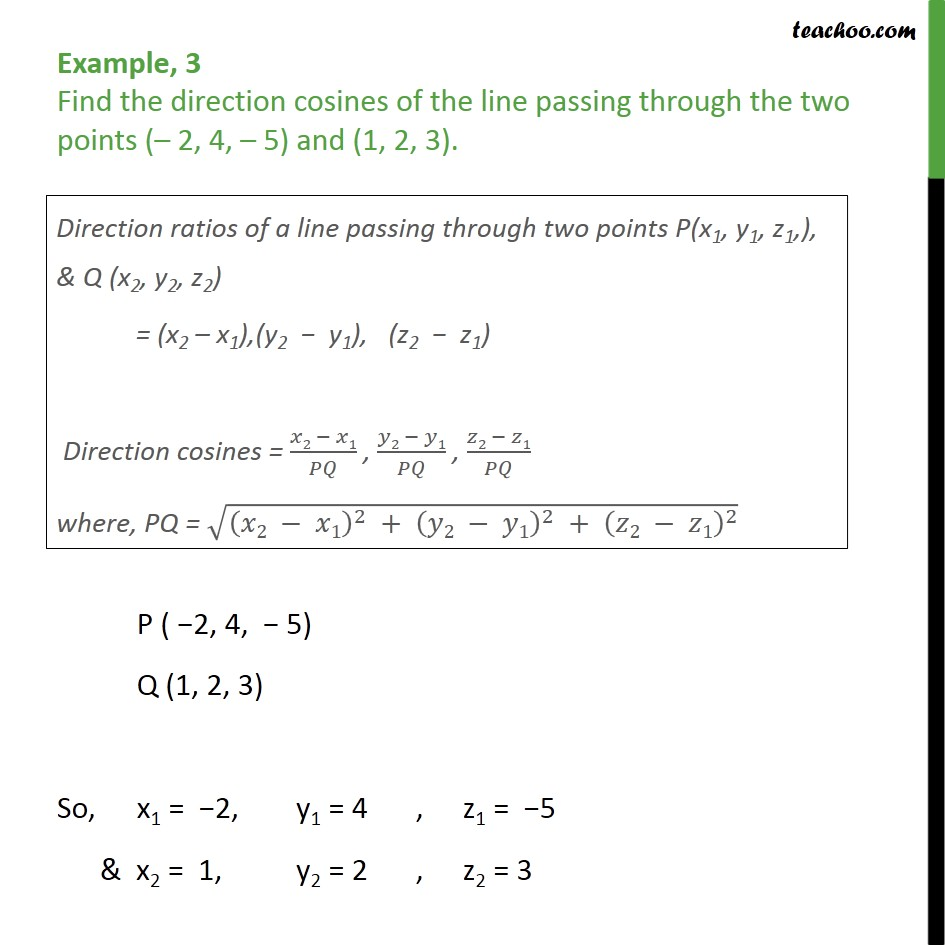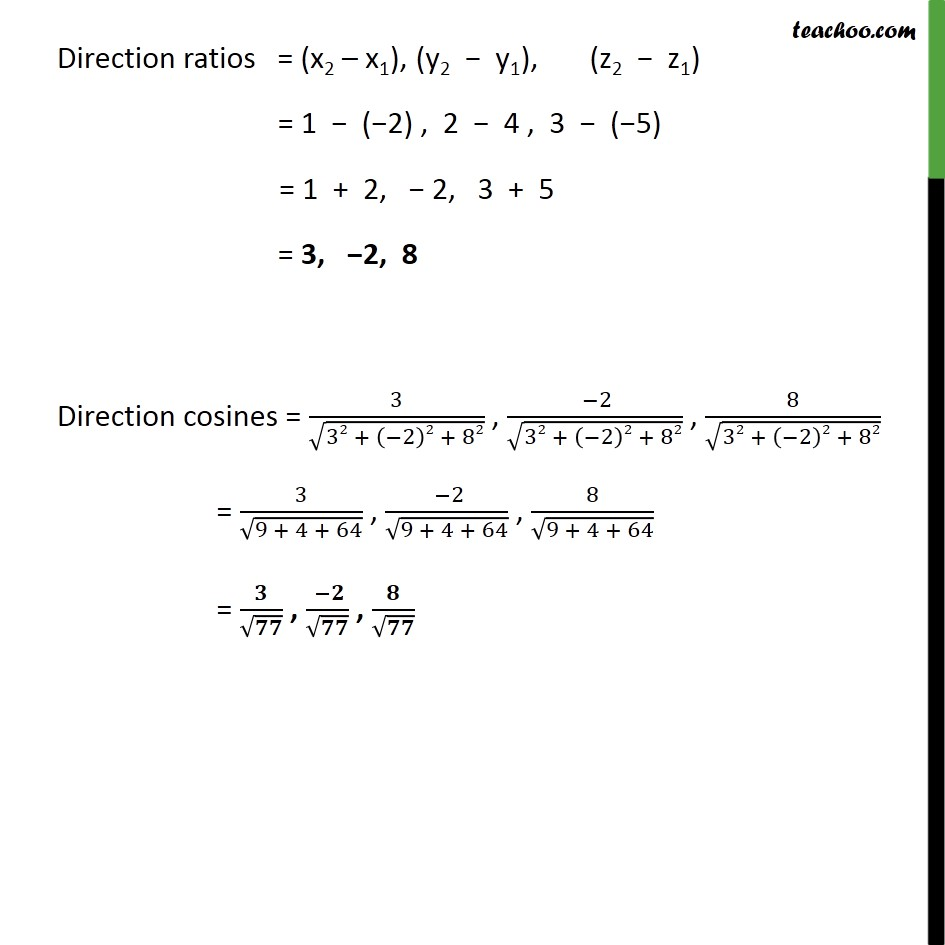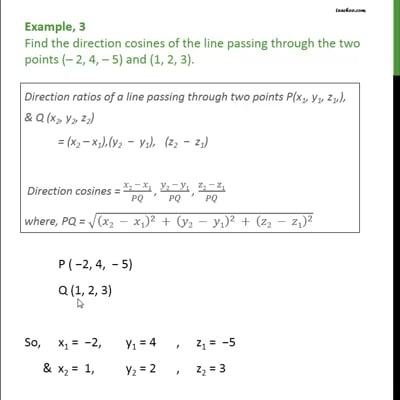Chapter 11 Class 12 Three Dimensional Geometry

Class 12
Important Questions for exams Class 12This video is only available for Teachoo black users

Learn in your speed, with individual attention - Teachoo Maths 1-on-1 Class

### Transcript

Example, 3 Find the direction cosines of the line passing through the two points ( 2, 4, 5) and (1, 2, 3). P ( 2, 4, 5) Q (1, 2, 3) So, x1 = 2, y1 = 4 , z1 = 5 & x2 = 1, y2 = 2 , z2 = 3 Direction ratios = (x2 x1), (y2 y1), (z2 z1) = 1 ( 2) , 2 4 , 3 ( 5) = 1 + 2, 2, 3 + 5 = 3, 2, 8 Direction cosines = 3 32 + 2 2 + 82 , 2 32 + 2 2 + 82 , 8 32 + 2 2 + 82 = 3 9 + 4 + 64 , 2 9 + 4 + 64 , 8 9 + 4 + 64 = , ,